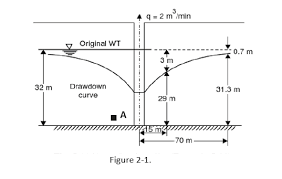## How to Calculate and Solve for Discharge Current | Unconfined Aquifer | Water BudgetThe image above represents discharge current.

To compute for discharge current, five essential parameters are needed and these parameters are Hydraulic conductivity (k), Elevate from impermeable to surface (H), Depth of water in the well (hw), Radius of influence (R) and Radius of well (γw).

The formula for calculating discharge current:

Q = πk(H² – hw²) / In[R/γw]

Where:

Q = Discharge Current
k = Hydraulic Conductivity
H = Elevate from Impermeable to Surface
hw = Depth of Water in the Well

Let’s solve an example;
Find the discharge current when the hydraulic conductivity is 10,the elevate from impermeable to surface is 4, the depth of water in the well is 20, the radius of influence is 5 and the radius of well is 10.

This implies that;

k = Hydraulic Conductivity = 10
H = Elevate from Impermeable to Surface = 4
hw = Depth of Water in the Well = 20
R = Radius of Influence = 5
γw = Radius of Well = 10

Q = πk(H² – hw²) / In[R/γw]
Q = π(10)[(4)² – (20)²] / In[5/10]
Q = 31.415[16 – 400] / In[0.5]
Q = 31.415[-384] / -0.693
Q = -12063.7 / -0.69
Q = 17404.26

Therefore, the discharge current is 17404.26.